top of page

## Interval Identification | Intervals of A Seventh (7 of 10)

So far in this video series we have learned how certain intervals have vibrational frequencies which are in greater harmony with each other and are in turn referred to as being Perfect, while other intervals can be either Major or minor depending upon the amount of steps separating each note.

Similar to an interval of a second, an interval of a third, and an interval of a sixth, an interval of a seventh can also be either Major or minor.

Let's start this video by discussing an interval of a minor seventh.

## Minor Seventh Intervals

An interval of a minor seventh occurs when two notes are separated by the distance of ten consecutive half steps.

Ten consecutive half steps can also be looked at as being four whole steps and two half steps.

If we followed this pattern of steps above the note A we would discover that an interval of a minor seventh above the note A is the note G.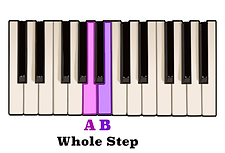One whole step above the note A is the note B.Another whole step above the note D is the note E.A half step above B is the note C.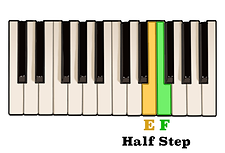A half step above E is the note F.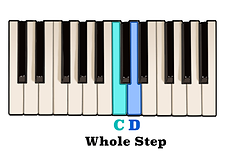The notes C and D are separated by a whole step.And finally, a whole step above the note F is the note G.

As you can see, an interval of a minor seventh above the note A is the note G.## Relation To The Scale | Minor Seventh Intervals

However, similar to what we had discussed about an interval of a sixth in the previous video, counting ten consecutive half steps can be time consuming and impractical and is not really the best approach when reading or writing an interval of a seventh.

We have already seen that a viable option for figuring out certain intervals above a given note would be to follow that note's corresponding Major or minor scale to the staff position which matches the interval that we are looking for.

Therefore, when trying to find an interval of a minor seventh above the note A, it is much more useful to follow the notes of an A minor scale until you reach the seventh scale degree. Here we can see that the seventh note in an A minor scale is in fact the note G.## Major Seventh Intervals

On the other hand, an interval of a Major seventh occurs when two notes are separated by the distance of eleven consecutive half steps.

When starting on the note C, eleven consecutive half steps would bring us to the note B.C and D are separated by one whole step.A whole step above the note F is the note G.D and E is also separated by a whole step.Another whole step above G is the note A.The notes E and F separated by one half step.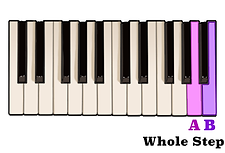And a whole step above A is the note B.

As you can see, an interval of a Major seventh above the note C is the note B.## Relation To The Scale | Major Seventh Intervals

However, like an interval of a minor seventh, the easiest way to determine what an interval of a Major seventh above any given note is would be to refer to that note's Major scale.

Here are the notes of a C Major scale. As you can see, B is the seventh scale degree and is therefore an interval of a Major seventh above the note C.Because of the distance separating each note, identifying an interval of a seventh on the staff can be a little confusing.

Although, when reading an interval of a seventh on the staff, you will notice that each note will either both be on a space, with three lines and two spaces between them,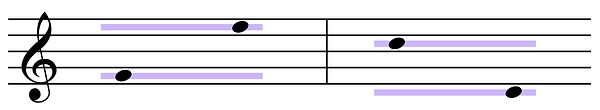or both on a line, with three spaces and two lines between them.bottom of page